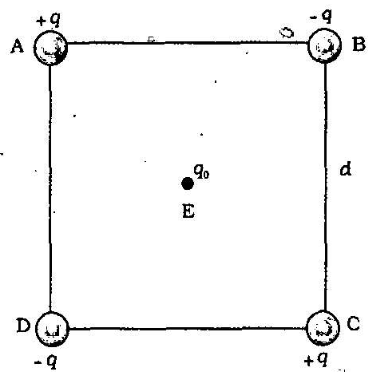Home/Class 12/Physics/

## QuestionPhysicsClass 12

Four charges are arranged at the corners of a square $$\mathit{ABCD}$$  of side d. as shown in the figure. Find the work required to put together this arrangement.Since the work done depends on the final arrangement of the charges. and not on how they are put together. we calculate work needed for one way of putting the charges at $$\mathit{A.}\mathit{B.}C$$ and $$D$$ . Suppose. first the charge $$+q$$  is brought to $$A$$ , and then the charges $$-q,+\mathit{q.}$$  and - $$q$$ are brought to $$\text B,\text C$$  and $$\text D$$ , respectively. The total work needed can be calculated in steps:
$$(i)$$   Work needed to bring charge $$+q$$ to $$A$$  when no charge is present elsewhere: this is zero.
$$(\mathit{ii})$$   Work needed to bring - $$q$$ to $$B$$  when $$+q$$  is at $$\mathit{A.}$$  This is given by $$($$ charge at $$\text B$$   $$\times$$    $$($$ electrostatic potentlal at $$\text B$$  due to charge $$+q$$  at $$\text A$$
$$=-q\times \left(\frac q{4\pi \varepsilon _0d}\right)=\frac{-q^2}{4\pi \varepsilon _0d}$$
$$(\mathit{iii})$$  Work needed to bring charge $$+q$$ to $$C$$ when $$+q$$ is at $$A$$ and $$-q$$ is at
$$\mathit{B.}$$ This is given by $$\{\left(\text{charge }\text{at}\text C\right)\times (\text{ potential at }\text C\text{ due to charges at }A\text{ and }B\;{\}}$$
$$=+q\left(\frac{+q}{4\pi \varepsilon _0d\sqrt 2}+\frac{-q}{4\pi \varepsilon _0d}\right)$$
$$=\frac{-q^2}{4\pi \varepsilon _0d}\left(1-\frac 1{\sqrt 2}\right)$$
$$(\mathit{iv})$$  Work needed to bring $$-q$$ to $$D$$ when $$+q$$ at $$A,-q$$ at $$\mathit{B.}$$ and $$+g$$ at $$\mathit{C.}$$ This is glven by $$\left(\text{ charge at }D\right)\times (\text{ potential at }D\text{ due to charges at }A,B\text{ and }C)$$
$$=-q\left(\frac{+q}{4\pi \varepsilon _0d}+\frac{-q}{4\pi \epsilon _0d\sqrt 2}+\frac q{4\pi \varepsilon _0d}\right)$$
$$=\frac{-q^2}{4\pi \varepsilon _0d}\left(2-\frac 1{\sqrt 2}\right)$$
Add the work done $$(i),(\mathit{ii}),(\mathit{iii})$$ and $$(\mathit{iv}):$$
The total work required is
$$=\frac{-q^2}{4\pi \varepsilon _0d}\left\{\left(0\right)+\left(1\right)+\left(1-\frac 1{\sqrt 2}\right)+\left(2-\frac 1{\sqrt 2}\right)\right\}$$
$$=\frac{-q^2}{4\pi \varepsilon _0d}\left(4-\sqrt 2\right)$$
The work done depends only on the arrangement of the charges. and not how they are assembled. By definition, this is the total electrostatic energy, of the charges. (Students may: try calculating same work/energy by taking charges in any other order they desire and convince themselves that the energy).